International
Tables for
Crystallography
Volume D
Physical properties of crystals
Edited by A. Authier

International Tables for Crystallography (2006). Vol. D, ch. 2.4, p. 329

## Section 2.4.2.1. Non-piezoelectric media

R. Vachera* and E. Courtensa

aLaboratoire des Verres, Université Montpellier 2, Case 069, Place Eugène Bataillon, 34095 Montpellier CEDEX, France
Correspondence e-mail:  rene.vacher@ldv.univ-montp2.fr

#### 2.4.2.1. Non-piezoelectric media

| top | pdf |

The fundamental equation of dynamics (see Section 1.3.4.2), applied to the displacement u of an elementary volume at r in a homogeneous material is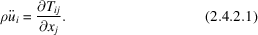Summation over repeated indices will always be implied, and T is the stress tensor. In non-piezoelectric media, the constitutive equation for small strains S is simply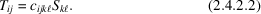The strain being the symmetrized spatial derivative of u, and c being symmetric upon interchange of k and, the introduction of (2.4.2.2)in (2.4.2.1)gives (see also Section 1.3.4.2)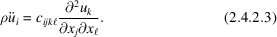One considers harmonic plane-wave solutions of wavevector Q and frequency ω,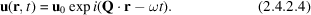For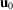small compared with the wavelength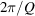, the total derivativecan be replaced by the partial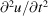in (2.4.2.3). Introducing (2.4.2.4)into (2.4.2.3), one obtains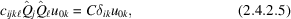where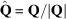is the unit vector in the propagation direction,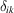is the unit tensor and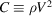, where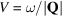is the phase velocity of the wave. This shows thatis an eigenvector of the tensor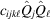. For a given propagation direction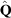, the three eigenvalues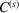are obtained by solving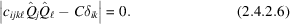To eachthere is an eigenvector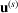given by (2.4.2.5)and an associated phase velocity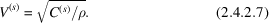The tensoris symmetric upon interchange of the indices (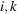) because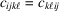. Its eigenvalues are real positive, and the three directions of vibration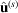are mutually perpendicular. The notationindicates a unit vector. The tensoris also invariant upon a change of sign of the propagation direction. This implies that the solution of (2.4.2.5)is the same for all symmetry classes belonging to the same Laue class.

For a general direction, and for a symmetry lower than isotropic,is neither parallel nor perpendicular to, so that the modes are neither purely longitudinal nor purely transverse. In this case (2.4.2.6)is also difficult to solve. The situation is much simpler whenis parallel to a symmetry axis of the Laue class. Then, one of the vibrations is purely longitudinal (LA), while the other two are purely transverse (TA). A pure mode also exists whenbelongs to a symmetry plane of the Laue class, in which case there is a transverse vibration with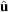perpendicular to the symmetry plane. For all these pure mode directions, (2.4.2.6)can be factorized to obtain simple analytical solutions. In this chapter, only pure mode directions are considered.